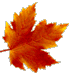HOME PAGE --------------- HELP+ » e-Design Notes --- » Switches, R, F » Resistors » Capacitors » Inductors » Diodes » Transistors --- » Potential Diff » Op Amps » Analog Filters --- » Boolean Algebra » Logic Gates » Flip-Flops » Logic ICs --- » Auto Injectors ---------------# ELECTRONIC DESIGN NOTES - CAPACITORSThe electronics world is ruled by the MOS [Metal Oxide Semiconductor] technology. That is, tiny pieces of silicon (or selenium/germanium), thin layers of metal (aluminum or copper) and even thinner layers of metal oxide (aluminum oxide or copper oxide).Silicon, germanium, selenium materials are enhanced with metallic impurities, therefore forming semiconductor electrical paths using induced electrical fields. The layers of metal create electrically conductive paths inside the tiny little chips, while the oxide layers act as insulators--and they are also needed to form . . . capacitors!

Capacitors are used generously in electronics since they have many (AC transient) functions. In this page capacitors are presented according to the following simplified structure:

1. Types of Capacitors
2. Useful Formulas
3. Equivalent Series and Parallel Capacitors
4. Growth and Decay in RC Circuits
5. Filtering Capacitors

NOTE

The basic notions highlighted in this page are related to a few electronic design topics presented in the first part, Hardware Design, of
LEARN HARDWARE FIRMWARE AND SOFTWARE DESIGN.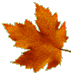1. TYPES OF CAPACITORS

In electronics, capacitors are used in principal to filter spikes and abrupt voltage variations; however, capacitors are good for many other things. Let's summarize what we can do with capacitors on our PCBs:

1. to filter EMI (induced Electro-Magnetic Interference);
2. to control the amount of reactance or inductance in AC circuits;
3. to build RC/LC timing circuits;
4. to control the "slew-rate" (the ramp) of electrical pulses;
5. to build resonant circuits;
6. to build frequency filtering circuits;
7. to build analog-to-decimal circuits;
8. to achieve coupling inductance;
9. to build SMPS (Switched-Mode Power Supply) power-pumps;
10. to store energy;
11. many more.

The most general classification groups capacitors as being:

1. fixed
2. variable

Considering the way they are inserted in electrical/electronic circuits, capacitors are:

1. through hole
2. surface mount
3. big capacitors, or batteries of capacitors having specially designed mechanical fixtures and connectors

A capacitor is made of two metallic plates positioned at a certain distance, and having a dielectric material in between. The nature of the dielectric influences capacitors' general construction. Therefore, considering their dielectric capacitors can be:

1. ceramic
2. mica
3. paraffin (plain, or with paper)
4. polyethylene
5. tantalum (metal and oxide)
6. electrolytic (ammonia)
7. air (vacuum)
8. glass
9. porcelain
10. oil (transformer oil)

Variable capacitors come in a few types, as follows:
1. RF variable capacitors
2. trimmers
3. banks of capacitors

NOTE
Although they are used a lot in DC circuits, please note that capacitors are AC-transient electrical components. As mentioned in Design Notes 1, there are no instances of pure DC circuits; all of them have some AC characteristics.2. USEFUL FORMULAS

In order to work with capacitors we need few mathematical formulas (as tools) close at hand. Here they are:

 CAPACITOR FORMULAS Formula Description C [F] = Q [C] / U [V] Capacitance K = C / Co (relative value; no unit) K = Dielectric constant C = Capacitance of the capacitor specific to the actual dielectric Co = Capacitance of the vacuum dielectric capacitor C [C] = (ε * A) / d C = Capacitance of the parallel plates type capacitor ε = dielectric permittivity A = Area of one plate d = distance between plates ε [C2/N * m2] = εo * K ε = dielectric permittivity εo = 8.85 * 10-12 [C2/N*m2] = permittivity of vacuum Z [Ω] = U [V] / I [A] Ohm's Law in AC circuits (details are presented in Design Notes 1) Z [Ω] = √[R2 + (XL - Xc)2] Impedance (details are presented in Design Notes 1) Xc [Ω] = 1 / (2 * PI * f * C) Capacitive Reactance 1 [pF] = 10-12 [F] Pico Farad 1 [nF] = 10-9  [F] Nano Farad 1 [uF] = 10-6  [F] Micro Farad

NOTE

In case you are unfamiliar, the notations within square brackets represent the unit. For example, Xc [Ω] means: Xc is measured in ohms. That is an important aspect, because we could have instances when the measurement unit is in mili-ohms, Xc [mΩ], or kilo-ohms, Xc [kΩ], therefore the formulas we use need to be scaled appropriately. For that reason the measurement unit must be added explicitly within square brackets.3. EQUIVALENT SERIES AND PARALLEL CAPACITORS

The equivalent of series resistors is calculated with:      1/CT =
Σ 1/Ci

The equivalent of parallel resistors is calculated with:     CT =
Σ
Ci

Calculation examples for series of three capacitors are presented next.Fig 1: The equivalent capacitance of 3 capacitors in series 1/CT = 1/C1+1/C2+1/C3 CT = C1*C2*C3 / (C2*C3 + C1*C3 +C1*C2) CT = 24 / (12+8+6) = 24 / 26 = 0.923 uF CT is smaller than the smallest serial capacitorFig 2: The equivalent capacitance of 3 parallel capacitors CT = C1+C2+C3 CT = 2uF+3uF+4uF = 9uF CT is greater than the greatest parallel capacitor4. GROWTH AND DECAY IN RC CIRCUITS

A capacitor in series with a resistor forms a timing circuit. This function is used a lot in electronics.

In RC circuits the time constant "T" is:          T [s] = R [Ω] * C [F]

In order to charge the capacitor to full capacity, however, it takes roughly 5 time constants (R*C) calculated with the above formula. The "decay curve" behaves perfectly similar to the "growth" one illustrated further down, having only an inverse second derivate (the curve holds water).

Fig 3: RC Charge Graph5. FILTERING CAPACITORS

This topic is described in Filter Design Notes #9.Take control of the extraordinary IT Revolution with Learn Hardware Firmware and Software Design--the easy way!» LINK TO "LOGICALLY STRUCTURED ENGLISH GRAMMAR"Page last updated on: July 25, 2018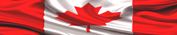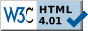Site pages valid according to W3C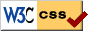Stylesheets pages valid according to W3C